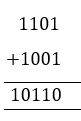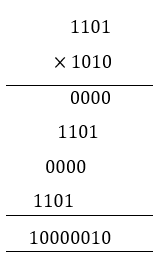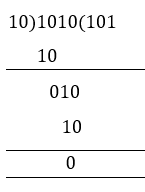# Binary Number System

A binary number system is one of the four types of number system. In computer applications, where binary numbers are represented by only two symbols or digits, i.e. 0 (zero) and 1(one). The binary numbers here are expressed in the base-2 numeral system. For example, (101)2 is a binary number. Each digit in this system is said to be a bit. Learn about the number system here.

Number System is a way to represent the numbers in the computer architecture. There are four different types of the number system, such as:

1. Binary number system (base 2)
2. Octal number system (base 8)
3. Decimal number system(base 10)
4. Hexadecimal number system (base 16)

In this article, let us discuss what is a binary number system, conversion from one system to other systems,  table, positions, binary operations such as addition, subtraction, multiplication, and division, uses and solved examples in detail.

## What is a Binary Number System?

Binary Number System: According to digital electronics and mathematics, a binary number is defined as a number that is expressed in the binary system or base 2 numeral system. It describes numeric values by two separate symbols; 1 (one) and 0 (zero). The base-2 system is the positional notation with 2 as a radix.

The binary system is applied internally by almost all latest computers and computer-based devices because of its direct implementation in electronic circuits using logic gates. Every digit is referred to as a bit

### What is Bit in Binary Number?

A single binary digit is called a “Bit”. A binary number consists of several bits. Examples are:

• 10101 is a five-bit binary number
• 101 is a three-bit binary number
• 100001 is a six-bit binary number
 Facts to Remember: Binary numbers are made up of only 0’s and 1’s. A binary number is represented with a base-2 A bit is a single binary digit.

## Binary Numbers Table

Some of the binary notations of lists of decimal numbers from 1 to 30,  are mentioned in the below list.

 Number Binary Number Number Binary Number Number Binary Number 1 1 11 1011 21 10101 2 10 12 1100 22 10110 3 11 13 1101 23 10111 4 100 14 1110 24 11000 5 101 15 1111 25 11001 6 110 16 10000 26 11010 7 111 17 10001 27 11011 8 1000 18 10010 28 11100 9 1001 19 10011 29 11101 10 1010 20 10100 30 11110

## How to Calculate Binary Numbers

For example, the number to be operated is 1235.

 Thousands Hundreds Tens Ones 1 2 3 5

This indicates,

1235 = 1 × 1000 + 2 × 100 + 3 × 10 + 5 × 1

Given,

 1000 = 103 = 10 × 10 × 10 100 = 102 = 10 × 10 10 = 101 = 10 1 = 100 (any value to the exponent zero is one)

The above table can be described as,

 Thousands Hundreds Tens Ones 103 102 101 100 1 2 3 5

Hence,

1235 = 1 × 1000 + 2 × 100 + 3 × 10 + 5 × 1

= 1 × 103 + 2 × 102 + 3 × 101 + 5 × 100

The decimal number system operates in base 10, wherein the digits 0-9 represent numbers. In binary system operates in base 2 and the digits 0-1 represent numbers, and the base is known as radix. Put differently, and the above table can also be shown in the following manner.

 Thousands Hundreds Tens Ones Decimal 103 102 101 100 Binary 23 22 21 20

We place the digits in columns 100, 101 and so on in base 10. When there is a need to put a value higher than 9 in the form of 10(n+1) for instance, to add 10 to column 100, you need to add 1 to the column 101.

We place the digits in columns 20, 21 and so on in base 2. To place a value that is higher than 1 in 2n, you need to add 2(n+1). For instance, to add 3 to column 20, you need to add 1 to column 21.

## Position in Binary Number System

In the Binary system, we have ones, twos, fours etc…

For example 1011.110

It is shown like this:

1 × 8 + 0 × 4 + 1 × 2 + 1 + 1 × ½ + 1 × ¼ + 0 × 1⁄8

= 11.75 in Decimal

To show the values greater than or less than one, the numbers can be placed to the left or right of the point.

For 10.1, 10 is a whole number on the left side of the decimal, and as we move more left, the number place gets bigger (Twice).

The first digit on the right is always Halves ½ and as we move more right, the number gets smaller (half as big).

In the example given above:

• “10” shows ‘2’ in decimal.
• “.1” shows ‘half’.
• So, “10.1” in binary is 2.5 in decimal.

## Binary Arithmetic Operations

Like we perform the arithmetic operations in numerals, in the same way, we can perform addition, subtraction, multiplication and division operations on Binary numbers. Let us learn them one by one.

Adding two binary numbers will give us a binary number itself. It is the simplest method. Addition of two single-digit binary number is given in the table below.

 Binary Numbers Addition 0 0 0 0 1 1 1 0 1 1 1 0; Carry →1

Let us take an example of two binary numbers and add them.

For example: Add 11012 and 10012.
Solution:### Binary Subtraction

Subtracting two binary numbers will give us a binary number itself. It is also a straightforward method. Subtraction of two single-digit binary number is given in the table below.

 Binary Numbers Subtraction 0 0 0 0 1 1; Borrow 1 1 0 1 1 1 0

Let us take an example of two binary numbers and subtract them.

Example: Subtract 11012, and 10102.

Solution: 1101– 10102 = 00112

### Binary Multiplication

The multiplication process is the same for the binary numbers as it is for numerals. Let us understand it with example.

Example: Multiply 11012 and 10102.

Solution:### Binary Division

The binary division is similar to the decimal number division method. We will learn with an example here.

Example: Divide 10102 by 102

Solution:### Uses of Binary Number System

Binary numbers are commonly used in computer applications. All the coding and languages in computers such as C, C++, Java, etc. use binary digits 0 and 1 to write a program or encode any digital data. The computer understands only the coded language. Therefore these 2-digit number system is used to represent a set of data or information in discrete bits of information.

## Problems and Solutions

Let us practice some of the problems for better understanding:

Question 1: What is binary number 1.1 in decimal?

Solution:

Step 1: 1 on the left-hand side is on the one’s position, so it’s 1.

Step 2: The one on the right-hand side is in halves, so it’s

× ½

Step 3: so, 1.1 = 1.5 in decimal.

Question 2:  Write 10.112 in Decimal?

Solution:

10.11 =  1 x (2)1 + 0 (2)0 + 1 (½)1  + 1(½)2

= 2 + 0 + ½ + ½

= 2.75

So, 10.11 is 2.75  in Decimal.

Keep visiting BYJU’S to explore and learn more such Math-related topics in a fun and engaging way.

## Frequently Asked Questions – FAQs

### What is a binary number system?

A number system where a number is represented by using only two digits (0 and 1) with a base 2 is called a binary number system. For example, 10012 is a binary number.

### What is a bit?

A bit is a single digit in the binary number. For example, 101 is three-bit binary numbers, where 1, 0 and 1 are the bits.

### How to convert a decimal number into a binary number? Give an example.

To convert a decimal number into its equivalent binary number, we divide the decimal number by 2 each time, till we get 0 as a dividend. Let us take an example to convert 1310 into a binary number.
13 ÷ 2: 6 and remainder 1
6 ÷ 2: 3 and remainder 0
3 ÷ 2: 1 and remainder 1
1 ÷ 2: 0 and remainder 1
Now we take the bits from the last remainder to first remainder, i.e.(MSB to LSB). Hence,
1310 = 11012

### What is the use of binary numbers?

Binary numbers are commonly used in computer architecture. Since the computer understands only the language of two digits 0’s and 1’s, therefore the programming is done using a binary number system.

### What is the value of 163 in binary?

The value of 163 in binary is 10100011.

### How is 200 represented in binary?

200 is the decimal number. The binary form of 200 is 110010002.

Quiz on Binary Number System